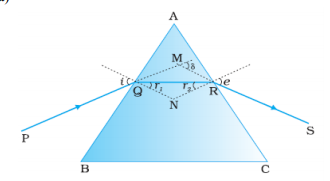(a) Draw the ray diagram showing refraction of light through a glass prism and hence obtain the relation between the refractive index $\mu$ of the prism, angle of prism and angle of minimum deviation.

(b) Determine the value of the angle of incidence for a ray of light travelling from a medium of refractive index ${\mu }_{2}=1$, so that it just grazes along the surface of separation.

5mark

(a)1/2 mark

From fig                                         1/2mark

From triangle

Hence from equ (1) & (2)

$\therefore \angle A={r}_{1}+{r}_{2}$                                                                               1/2mark

The angle of deviation                                                                               1/2mark

$\delta =\left(i-{r}_{1}\right)+\left(e-{r}_{2}\right)=i+e-A$

At minimum deviation i=e and ${r}_{1}={r}_{2}$                                                         1/2mark

$\therefore r=\frac{A}{2}$                                                                  1/2mark

And $i=\frac{A+\delta m}{2}$

Hence refractive index

1/2mark

(b) From Snell's law

Given

1/2mark

or                                                                      1/2mark

Difficulty Level: